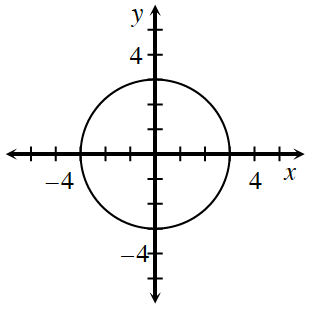### Home > GC > Chapter 12 > Lesson 12.1.3 > Problem12-26

12-26.Examine the graph of the circle at right.

1. Find the equation of the circle.

Where is the center of the circle?
What is the radius of the circle?

2. On graph paper, sketch the graph of the equation $x^2 + y^2 = 49$. What is the radius?

Use the eTool below for help with this problem.
Click the link at right for the full version of the eTool: 12-26 HW eTool